# Calculate the return on investment on stock##### CALCULATING YOUR RETURN OVER TIMEToday we will be going through 3 approaches to calculate your Return on Investment, or your portfolio return so you can truly understand what you are getting either this year, or from multiple years in your portfolio.

What surprises me most is that many people will teach you about the first and second approach, but I have never seen anyone mention the third approach, which is probably the most complicated yet most accurate method.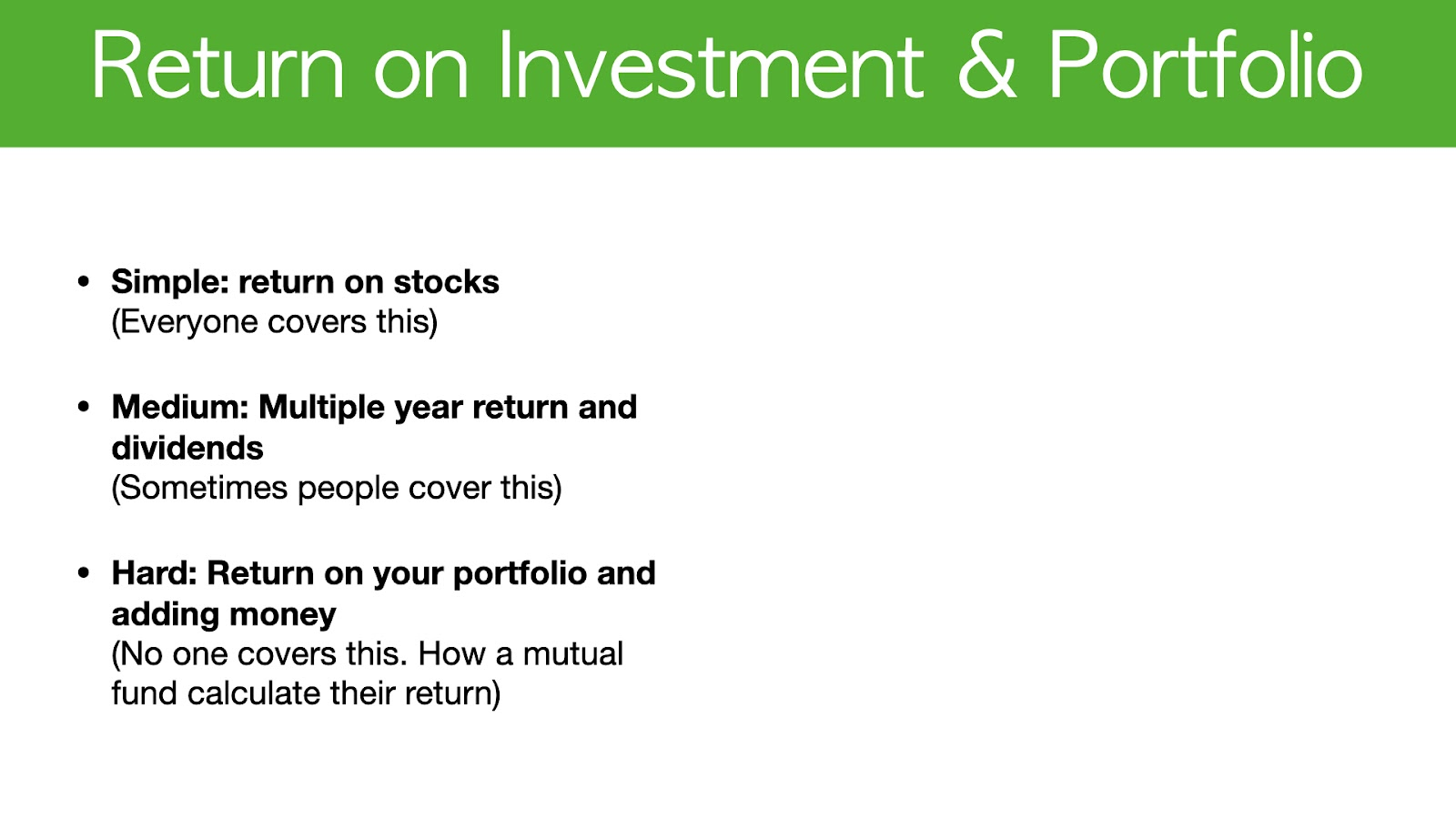The first approach is simple - You can find this method on how to calculate your return on stocks through a quick Google or YouTube search.

The second approach is to calculate multiple years of return, including dividends. I have seen a few online resources that will cover this topic at a relatively advanced level, so you will be able to find this method online as well.

The third method is to calculate the return on your portfolio, including adding money.

This is probably the most important method thus far because adding money will affect your investment return.

So far, I haven’t found any resources that will show you how to calculate your ROI with added money. In fact, this is actually how a mutual fund calculates the fund return.

Before we start, I just want to let you know that the absolute best way to calculate your own return and skip all the math is to choose a broker that will do the calculation for you.

#####So let’s jump into my own portfolio. My broker is TD Canada, and now I will show you how they calculate my return.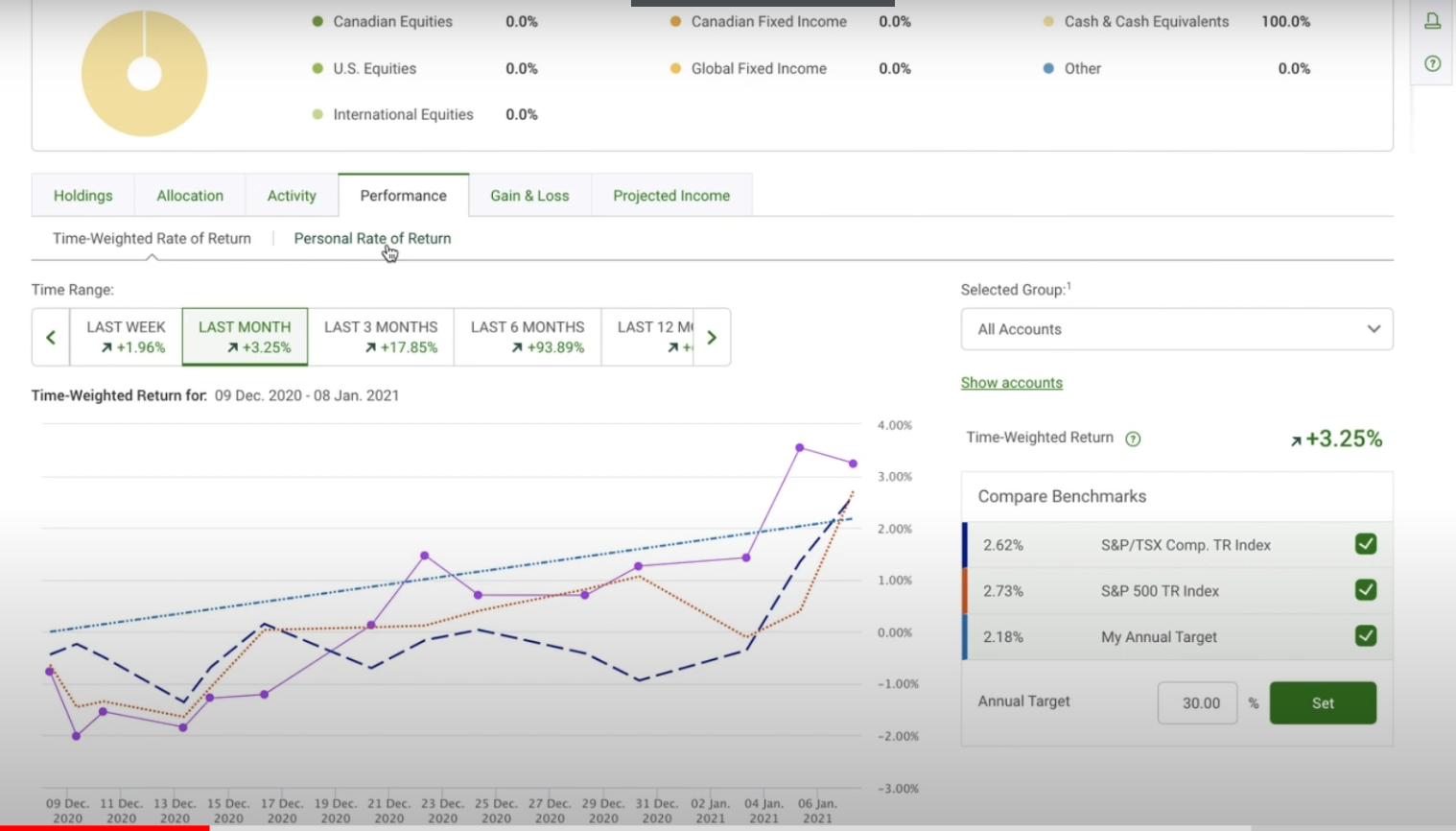So I have logged into my portfolio and if you have TD Canada, you will find this under the “Performance” tab and I use “time-weighted return”.

At this point, you can select the period of time you want to view. For myself, I like to look at the long-term, so I will scroll on this bar here and choose “since inception”.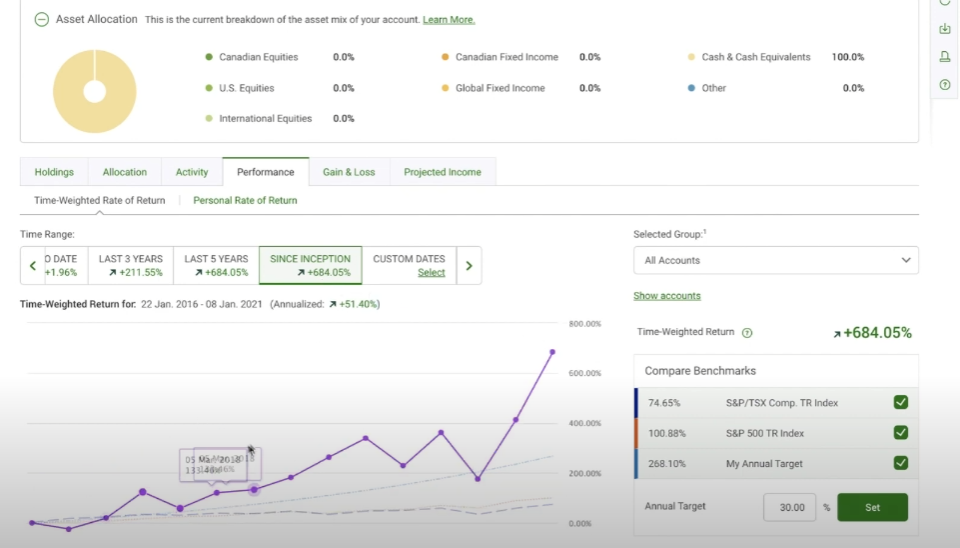This will probably give you the best idea on what your return is.

Afterwards, on the right-hand side you will select “All accounts”.

In this case you can see that for my TFSA and RRSP account, my time weighted return which excludes any influence of adding capital in, is 684%.

Afterwards, it also calculates my annualized return to be 51.4% which is very helpful. This way, you won’t need to go through all the calculations yourself.

However, if you are like me and have multiple brokers or multiple accounts and need to calculate this return yourself, then the rest of this post is dedicated to you.

When looking at your return, you are also able to compare with a target that you set, for example, I set my target ta a 30% return indicated by the blue line.

You also get to compare with the S&P 500 and also the Toronto Stock Exchange composite index. These two are the red lines and the dashed blue line you see below.

So my portfolio started in January 2016 and now it is currently January 2021.

#####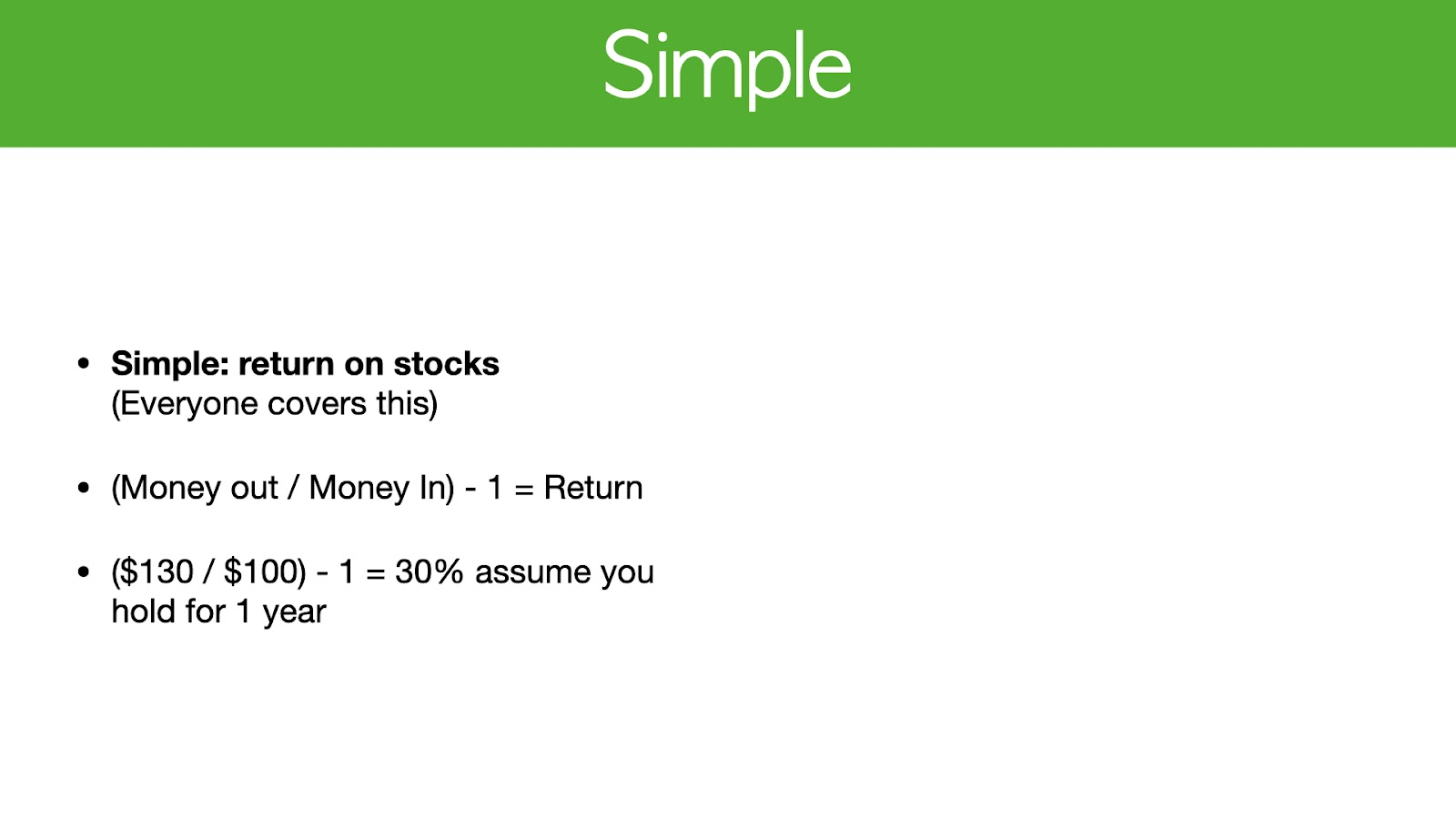The first simple method for calculating return is basically the money you get divided by the money you put in, minus 1. Nice and simple.

Here is an example: You invest \$100 in Google.

At the end of the year, you get \$130.

In this example, the market value is \$130 / \$100 - 1 which gives you 0.3 or 30%.

This assumes that you hold for one year.

Obviously this is an accurate calculation for a single year, but if you do this across multiple years and you divide it by let’s say five years, then the return you calculated will not give you the compounded figure.

However, this method remains the easiest way to calculate a one-year return.

#####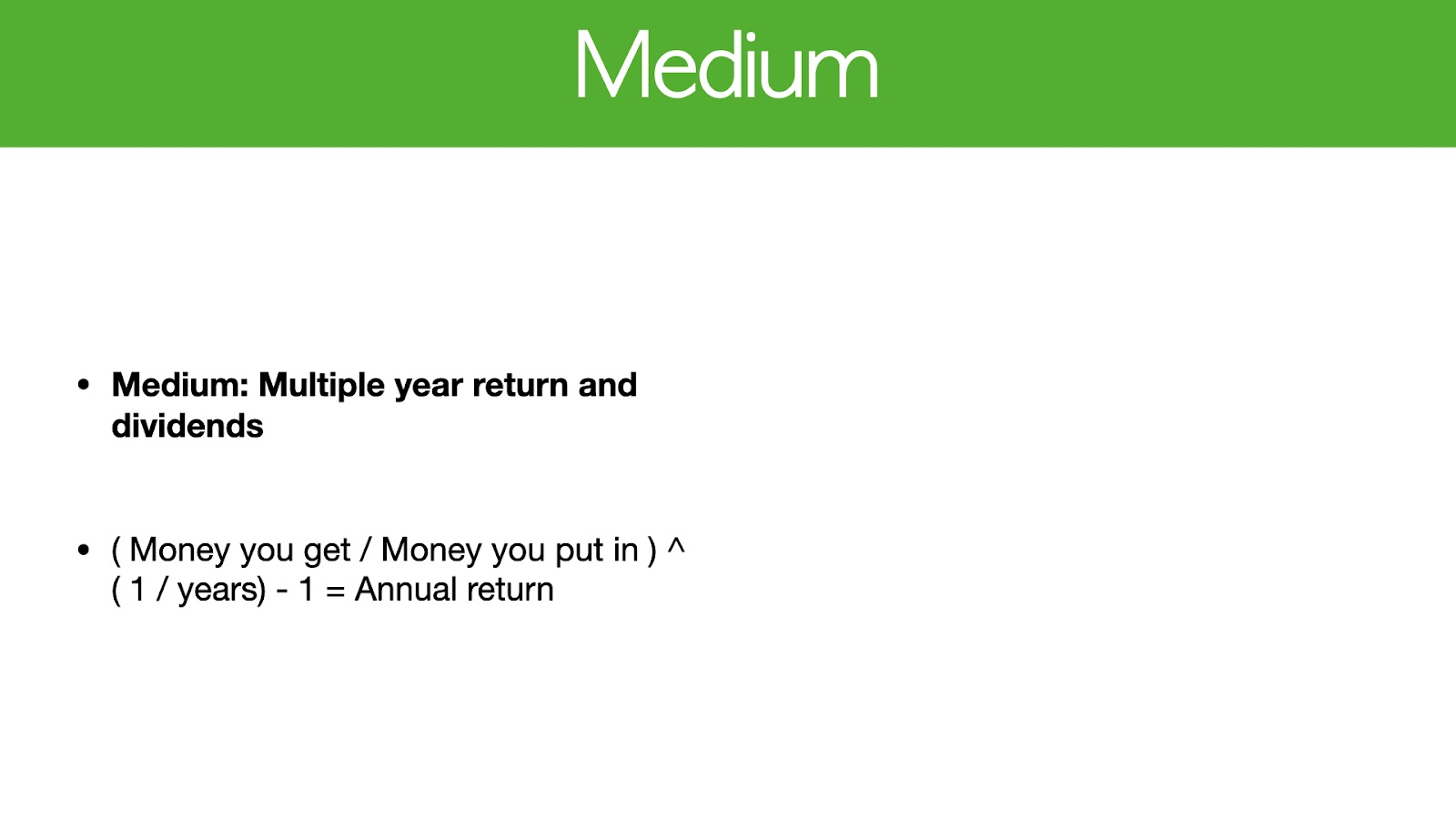The medium approach includes dividends and multiple years of return, and the formula to calculate this is listed above.

Here is an example of this calculation:

At the start of 2021, you put in \$100 and get a dividend of \$10.

You also sell the investment for \$150, so you have a gain on investment and also a dividend.

Let’s say you sold it within 180 days, or half a year.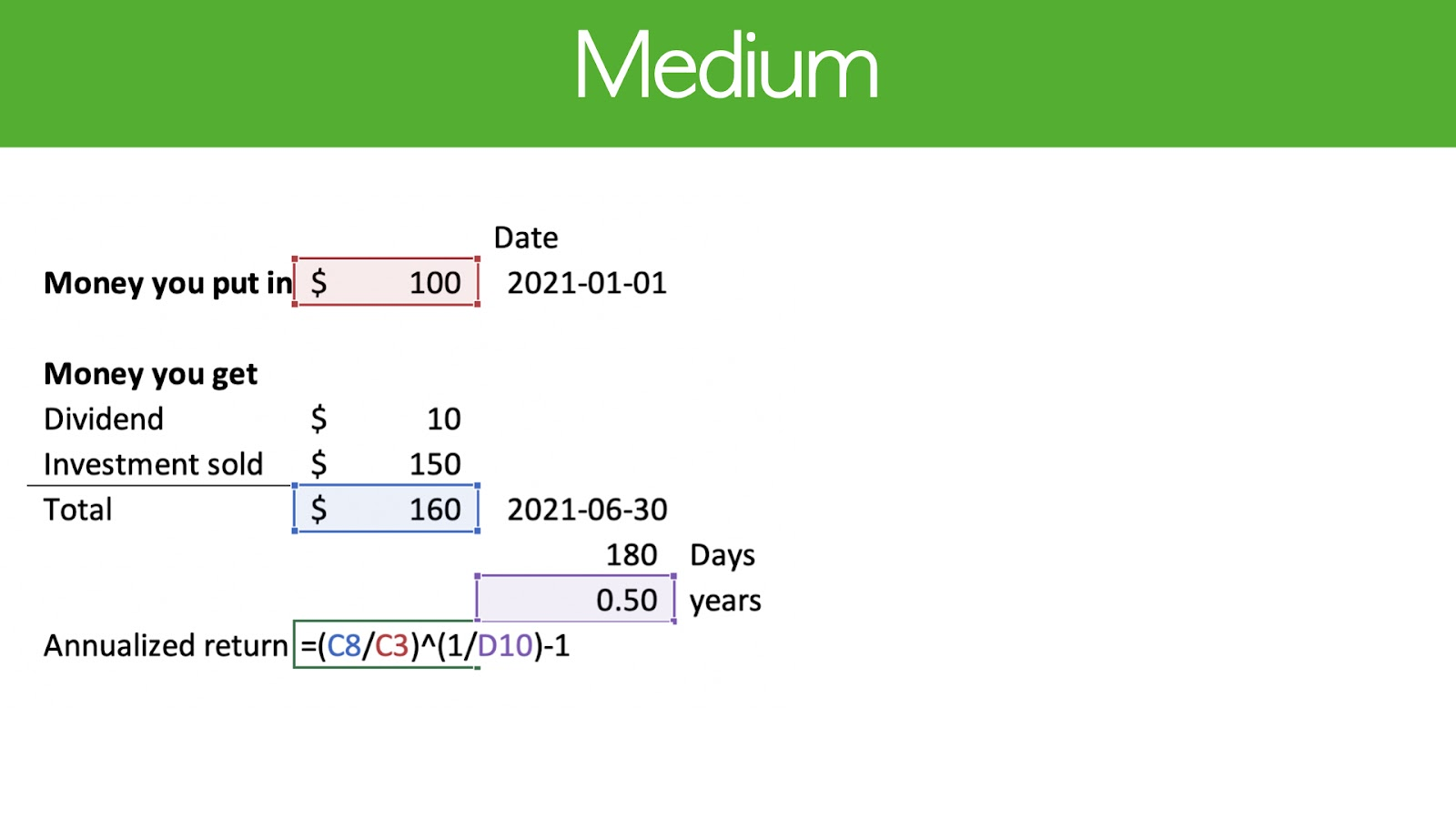Here are the numbers plugged into the formula from an excel sheet.

If you want to replicate this within the Excel sheet that you are using to calculate your portfolio return, then you can do so now by taking a few minutes to study this formula.

As you can see, this is a very straightforward equation and a fairly simple way to calculate your return.

#####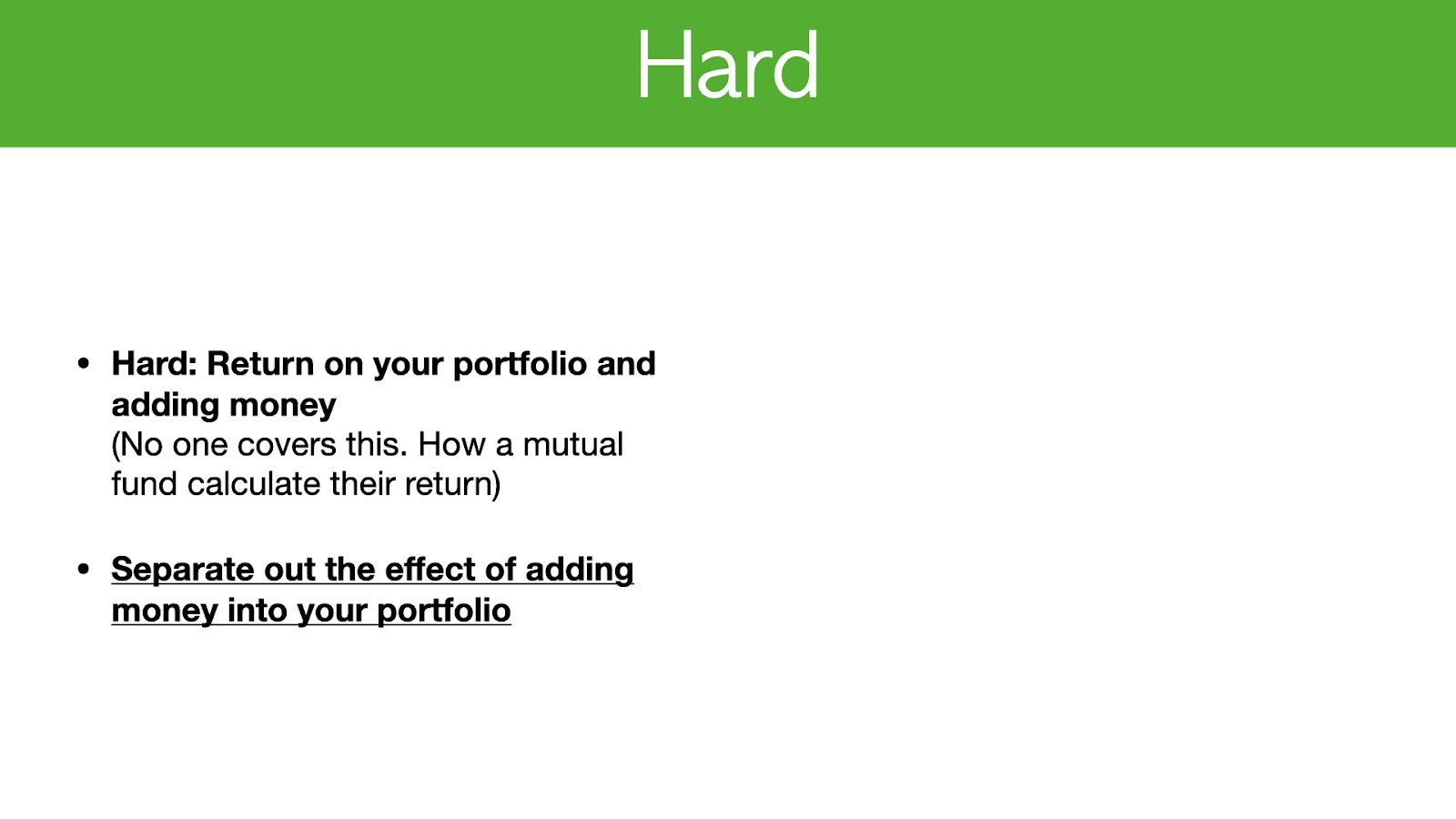When you put money into your portfolio, this influences your return and this is why a third approach is required to accurately calculate your investment return.

This is the most difficult yet most accurate calculation and it is also how mutual funds calculate their returns. It basically isolates the effect of adding money into your portfolio.

Firstly, let’s talk about why it’s so important to separate the effect of adding money into your portfolio.

I’m going to show you first the incorrect way to separate the effect of adding money into your portfolio and how this will influence your return so you can better understand how to do it correctly.

#####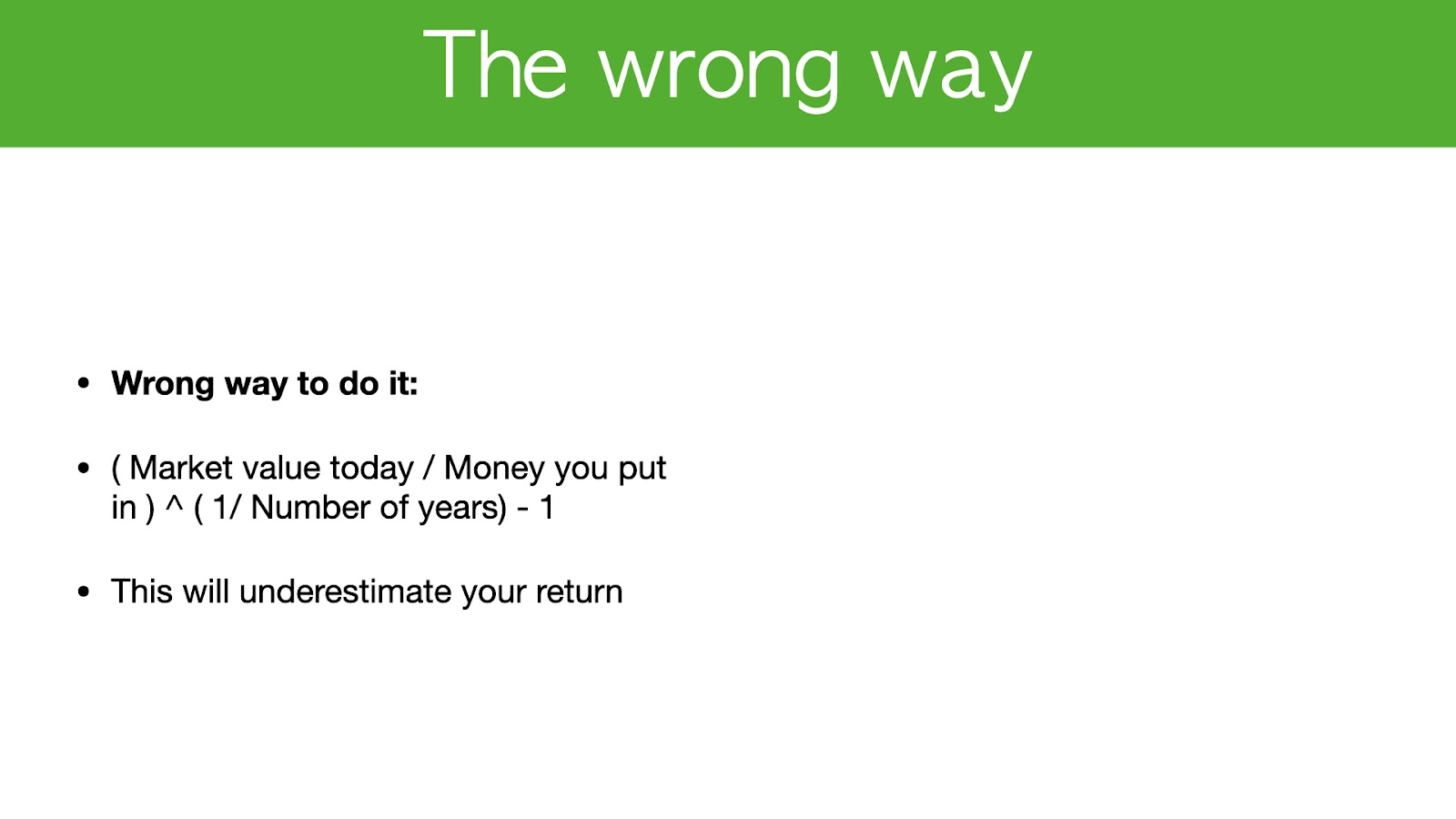The wrong way to do it is to divide the market value today by the amount of money you put in, regardless of which time you put it in, to the power of one divided by the number of years minus one.

Doing this will actually underestimate your return and I have provided an example for you.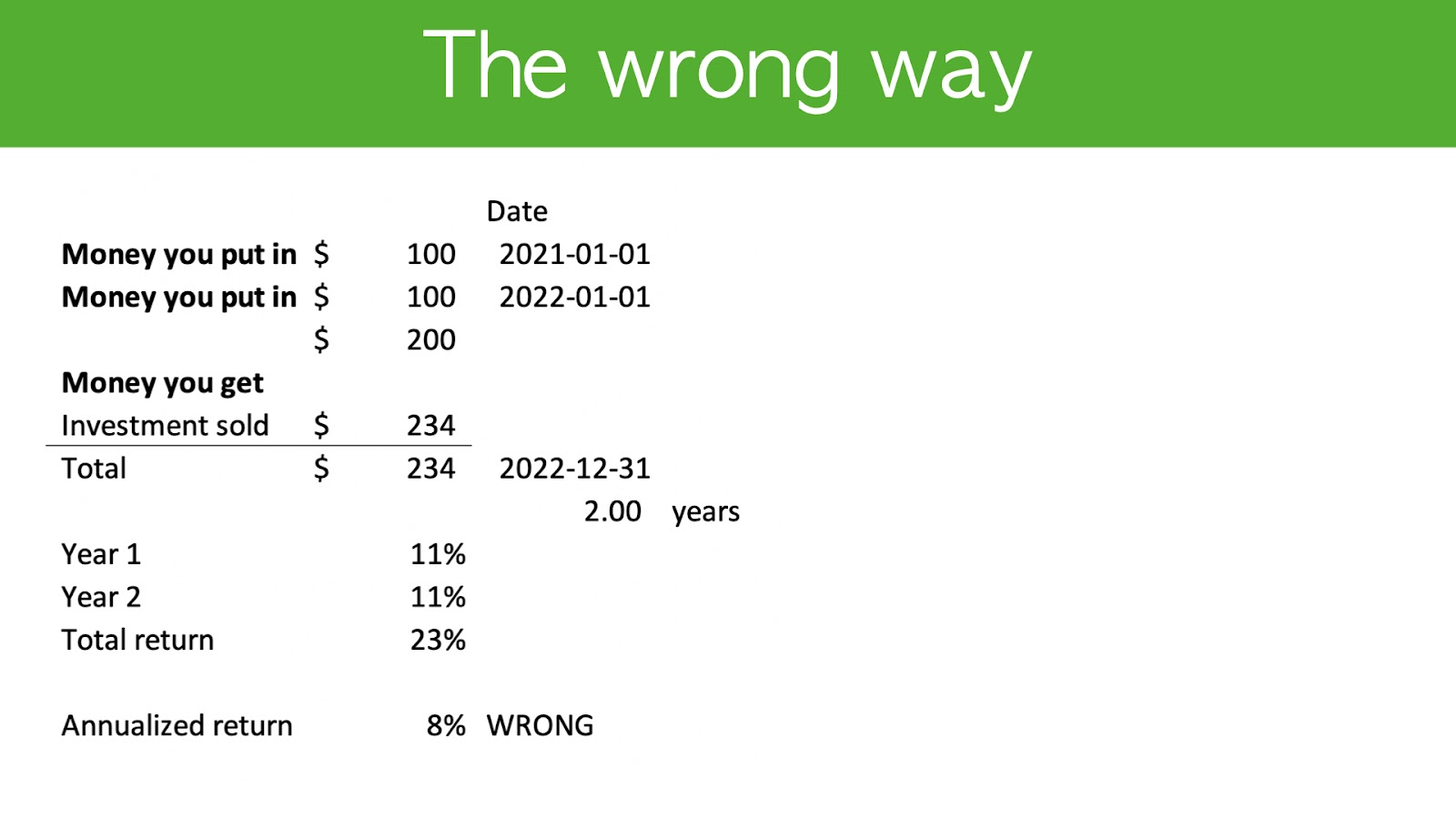In this example, you put \$100 into a stock like Google.

The next year you also put in \$100 into the same stock and by the end of the second year, you sell it for let’s say, \$234.

In year 1, the stock grows by 11% and in year 2 the stock also grows by 11%.

If you try to calculate the investment for your first \$100 it grows by 23% and your investment for the second \$100 should grow by another 11%, which is how you get \$234.

Now when you think about your annual return and use the formula I’ve shown you, it actually assumes you invested all the money in the beginning of year 1, even though \$100 is from year 2.

Based on this calculation, it will give you an annualized return of 8% which is incorrect because the gain was actually 11% in year 1 and another 11% in year 2, therefore the gain should be 11% for this portfolio.

In order to fix that, you’ll need to separate out the effect of adding money into your portfolio, and this is how a mutual fund or hedge fund does it.

#####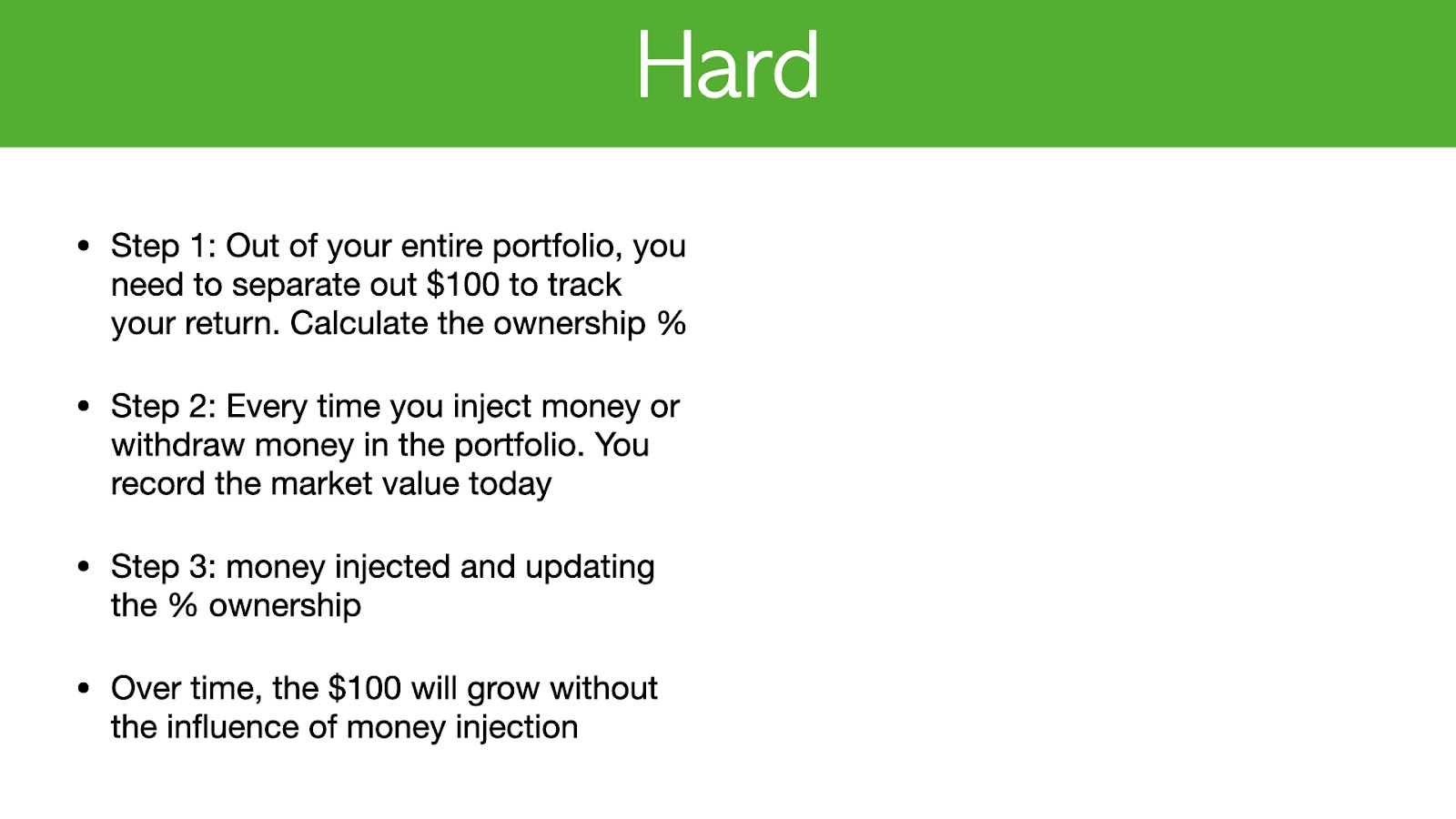First out of your portfolio you’ll need to separate \$100 to track your return and calculate it from an ownership percentage.

Then the next step would be to record the market value of today every time you insert or withdraw money from your portfolio.

For step 3 you will add the money, add this to your portfolio and update the ownership percentage.

By doing so, over time your \$100 will not be influenced by the amount of capital you put in at later times, and you will be able to calculate your true return over multiple years.

Here’s an example of how it works.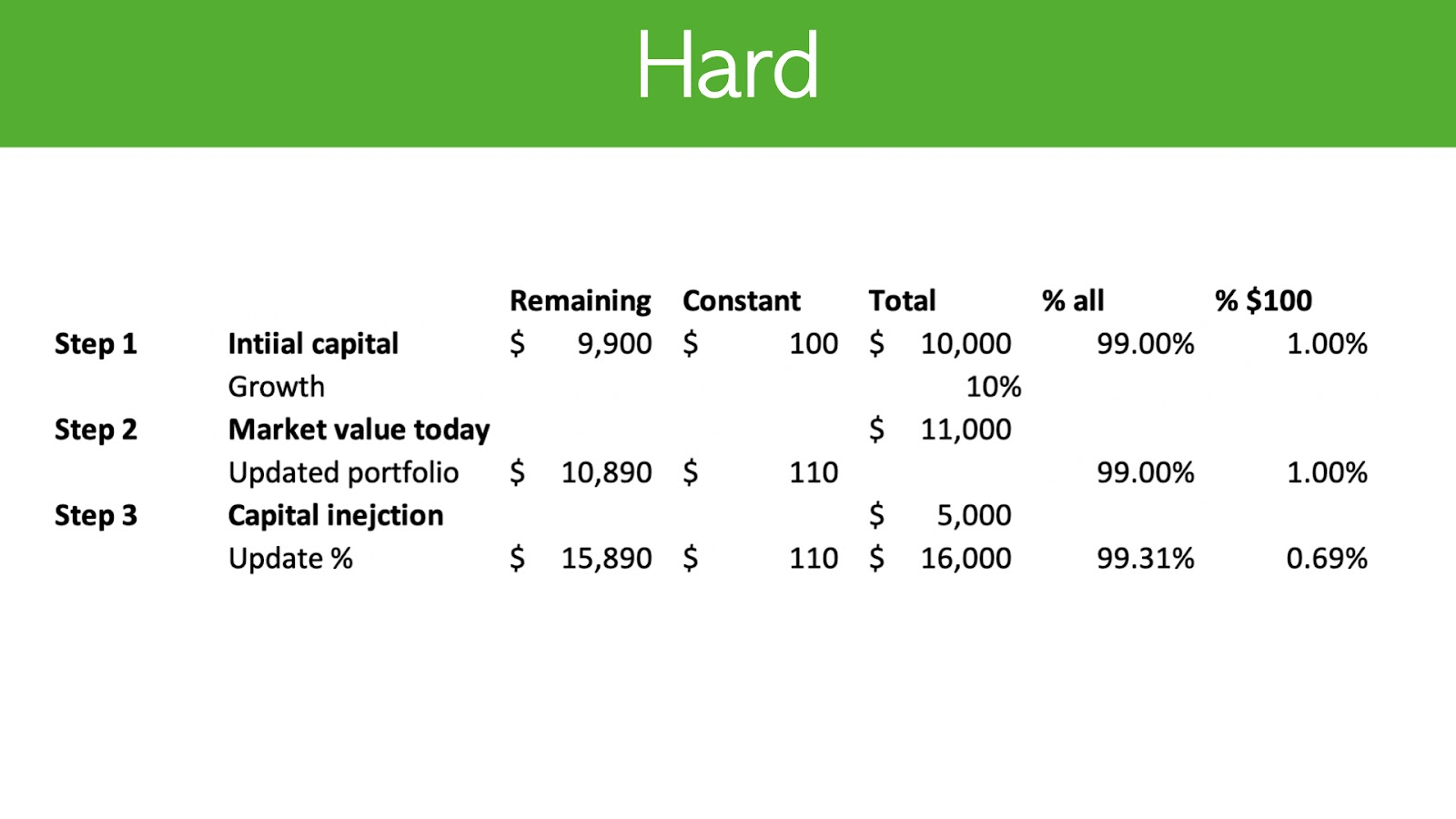In Step 1, you’ll see that there is a total or \$10,000 in the portfolio.

In the “less” column you will see that there is a remaining balance of \$9,900 because I’ve separated \$100 out as a constant, and I’ve also expressed these figures as a percentage as well.

So you can enter in whatever amount and also express your figures as a percentage.Step 2 shows the market value of your portfolio today.

If my portfolio has grown by 10%, then it would now be \$11,000.

My \$100 becomes \$110 and my \$9900 becomes \$10,890.

If at this point you decide to insert capital by adding \$5,000 into your portfolio, that would bring your remaining portfolio to \$15,890.

Observe that the constant remains at \$110. This means that injecting capital did not influence your return because it all went to the remaining column.

At this point, you will take the total which is \$16,000 and recalculate the percentage.On the very last line, you will see that the figure is now 99.31% and the constant percentage becomes 0.69%. This is exactly how a mutual fund would calculate it.

As you can see, you will need to know the market value every single time you decide to put money in. This is a crucial part in the accuracy of your calculation on returns.

If you do not want to do all of this, I would recommend that you get a broker to do it for you.

# 90% correct transcript

So Linda's video, I'm going to go through three approaches to calculate your investment return, your portfolio return. So then you truly understand what is the return you're getting for this year or multiple years for your portfolio. And the surprising thing is most people cover the first or the second approach, but I haven't seen any one covered a third approach. And that is probably the hardest and also the most accurate. And the third approach is actually how a mutual fund does their accounting and calculate dare return. So make sure you stay till the end. So then you really understand how to calculate your own return over time. So let's get started 96.4% of you guys are not subscribed yet. So it would be a great help. If you can just tap that subscribe button, it is free and you can always change your mind in the future.

Make sure you stay till the end for the giveaway as well. And investing a celebrator. I just want to celebrate the 99th case study where Carolyn made 20% from shake shack in nine months. So congratulations, Carolyn. And this month I'm looking to help. 20 professionals will photo financial background to master investing and target 30% a year. The stretch goal is 30 people, and there'll be more details at the end of the video. So the first simple approach is really calculating your return on stocks and everyone pretty much covers this and you can find it on YouTube. Now the second approach is really to calculate multiple years of return, including dividends. Now, sometimes I've seen some videos cover this at a relatively high level. Now the third one is the return on your portfolio, including adding money. And this is probably the most important because adding money does affect your investment return.

And so far, I haven't seen anyone actually covers this, and this is actually how a mutual fund calculate the fund return. Before we even start. I just want to say the best way to calculate your own return and to skip all the math is to choose a broker Dow due to calculation for you. So I'll jump into my portfolio, my broker, which is TD Canada, and I'll show you how they calculate my return right now. Now here, you actually find my TD Canada log-in screen. We're actually logged into my portfolio and it is Ashley under accounts. And if you have TD Canada and it's under underperformance and it will actually bring you to this screen. So when it comes to finding your investment return, it's under the performance tab and I use time weighted return. That is the one you're looking for, the personal rate of return.

I don't know how to calculate it, but that is not what you're looking for. So time-weighted to return. And then afterwards you can choose to period. So for myself, I really like to look at the long-term. So I would actually go all the way over here and choose since inception. And that will probably give you the best idea on what your return is. And then afterwards you would choose all accounts. So in this case, you can see that for my TSA and my RSP account, my time weighted return, which excludes any influence of adding capital in is 684%. And then afterwards it also calculates my annualized return to be 51.4%. So that's actually very helpful. So you don't need to go through all the calculations yourself, but if you're like me, where you have multiple brokers or multiple accounts, and you need to calculate this return yourself, then the rest of the video is for you.

Now, when it comes to looking at this return, you also get to compare with a target that I set, which is for example, a 30% return. And this is the blue line here. And you also get to compare with SMP 500 and also Toronto stock exchange composite index. So these two are the red line and the dash blue line you see below. So my portfolio started really January, 2016 and now is January, 2021. So first the simple method for the simple method to calculate in investment return is basically the money you get defeated it by the money you put in very simple minus one. So let's take an example. You invest in Google a hundred dollars. And at the end of the year, you get \$130. So the market value is 130 divided by a hundred. And then afterwards you minus one. So that gives you 30%.

And this assumes you to hold for one year. And obviously this is accurate when it is one year, but if you do this across multiple years and you defied it, let's say five years, then the return are calculated is actually not compounded, but this is the easiest way to calculate a one-year return. So we're going to breeze through that and go to the medium approach now to medium approach includes dividends and multiple years of return. And the formula is the money you get, which includes the market value. As of today, plus the dividend, you get divided by the money you put in, and then you would tick that one, divide it by the number of years. And this will give you your annual return per year for multiple years. So let's go through an example. So let's say in the beginning of 2021, you put in a hundred dollars and you get dividend of \$10 and you also sold the investment for \$150.

So you have a gain on investment and also your dividend. So let's say you sold it within 180 days. So it was half a year. So when you think about that, you would take her one 60 divided by 100 to the power of two and then minus one. So the next slide I'll actually show you the formula and you can see the blue cell C eight divided by C3, the red cell, and then to the power of one divided by the purple South, which is 0.5 minus one. So if you want to replicate this within the Excel that you're using to calculate your portfolio return, then you can do so now by pausing this video and studying this formula, and this is a fairly simple way still to calculate your return. And when you put money into your portfolio, that actually influences your return as well.

And that's why you need to third approach to calculate your investment return. Now, this is really the hard method and this is the most accurate method. And this is actually how a mutual fund calculates their return. And this basically separates out the effect of adding money into your portfolio. Now you might ask why is it so important to separate the effect of adding money into your portfolio? So I'm going to first show you the incorrect way of doing it and what happens if you don't do it the hard way and how that influence your return. So then you better understand how to do it correctly. So first the wrong way. So the wrong way to do it is really the market value today divided by the amounts of money you put in, regardless of which time you put in to the power of one divided number of years minus one.

So this will actually underestimate your return. And here I have prepared an example for you. So let's say this year, you put a hundred dollars into a stock. You like Google. And the next year you also put in a hundred dollars into the same stock. And by the end of the second year, you sell it and let's say you sell it for \$234. And the way I come to this \$234 is that's in year one. The stock grew by 11% and in year two, the stock grew by 11%. So if you try to calculate, you know, D investment for your first \$100, it grew by 23% and your investments for a second \$100 should grow by another 11%. Then that's how you get to the two 34. Okay. Now, when you are thinking about your annual return and you'd use the formula, I just showed you there.

It actually assumes you invested all the money in the beginning of year one, even though \$100 is in year two. So that will get you an annualized return of 8% you see here, and you know, that is wrong because in year one, the game was 11 and in year two, the game was 11 as well. So the annual return should be 11% for this portfolio. And in order to fix that, you'll need to separate out the effect of adding money into your portfolio. And this is how a mutual fund or a hedge fund does it. So first out of your portfolio, you need to separate out, let's say a hundred dollars to track your return and you need to calculate an ownership percentage. And for step two, every time you inject money or you withdraw money from your portfolio, you need to record the market value today.

And then step three, you add the money, you inject it into the portfolio and then update the ownership percentage. And then over time, your \$100 will not be influenced by the amount of capital you put in. So then you can calculate your true return over multiple years. So let's see an example and see how that works. So here you'll see the table and step one, I put in \$10,000 in the portfolio, and here you will see the total column, which says \$10,000. And the less column you will see remaining \$9,900 because I've separated out a hundred dollars as a constant. So that is relatively straightforward. And when you're trying to calculate the percentage Yossi that's 99% is all, and 1% is considered to be the 100. So if you're investing in a portfolio that has a hundred thousand or a million, you just do the same thing, just separate out a hundred dollars for tracking purposes.

Now, step number two is really the market value today. And let's say my portfolio grew by 10%. So now as \$11,000. So what I'll do is using the same percentage I calculated in step one, I'll apply that and calculate the market value today for my \$100 and also to \$9,900. So it turns out my \$100 grew by 10%. So it was 110, and that makes sense. And then the remaining portfolio grew to \$10,890. Okay. So you're following me so far. And at this point, you then inject capital by adding \$5,000 into your portfolio. So that brings your remaining portfolio to \$59,890. And what you will observe here is actually the constant remains at \$110. So that means injecting capital actually did not influence your return because it all went to the remaining column. And at this point, you'll take the total, which is 16,000 and you'll recalculate the percentage.

So on the very last line, you will see that it is 99.3, 1% now, and the constant percentage becomes 0.69%. And that is exactly how a mutual fund would calculate it. And as you can see the steps you'll need to know the market value every time you put money in. And that is very crucial because that's actually gets you the most accurate return. So if you don't want to do all of this, that is better to get a broker out, do it for you. Um, and for myself, I use TD Canada when I'm investing. My vision for myself is really to get 30% a year. And this is like going to Mars initially because 30% is such a stretch goal. And that's why I put together a free four hour training on how I mastered investing and how I've gone through from a person who's losing money year after year to a person who can make 30% a year.

So this is perfect for you. If you're a full-time professional with no financial background, and you want to learn how to manage your own portfolio with confidence. So the link is in the description is five minute investing.com/free case study. And it outlines my journey from going to a loser losing more than 10% a year to making 30% a year using a simple, proven and effective investing strategy. So it took me eight years to figure this out on my own. So if you're new to investing, definitely check it out. And it is quite long because I put a lot of content in it and it will put you miles ahead of consuming another 100 YouTube videos. And throughout my coaching career, I have helped Carolyn to make 20% from shake shack at nine months. So that is the 99th case study within investing accelerator. And this month I'm looking to help 20 professionals without a financial background to master investing and to target 30%.

That stretch goal is 30 people. So if you attend the free case study in the first link of description, and you can schedule a call with me to chat, to see if you're a good fit now, in terms of the giveaway, I'm planning to give away another copy of Sam Walton made in America. So if you just take a minute to like the video, leave a comment below and I'll announce the winner and one to two months. So the winner from a previous video is Fred, and he wanted to book harmonic trading too. So Fred, if you're watching this video, then make sure you send me your details and I'll send a book your way. So that is pretty much it for this video. And I'll see you in the next one.

#### Eric Seto

Investor, CPA (Canada) based in Hong Kong and Vancouver